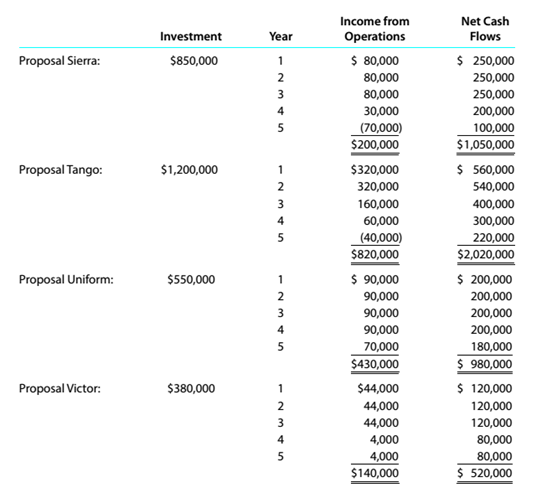Chapter 15, Problem 15.6.2P

Chapter
Section
Textbook Problem

Capital rationing decision involving four proposalsKopecky Industries Inc. is considering allocating a limited amount of capital investmentfunds among four proposals. The amount of proposed investment, estimated income fromoperations, and net cash flow for each proposal are as follows:The company’s capital rationing policy requires a maximum cash payback period of three years. In addition, a minimum average rate of return of 12% is required on allprojects. 1f the preceding standards are met, the net present value method and presentvalue indexes are used to rank the remaining proposals. Instructions Giving effect to straight-line depreciation on the investments and assuming no estimated residual value, compute the average rate of return for each of the four proposals. Round to one decimal place.

To determine

Concept Introduction:

ARR:

Accounting Rate of Return (ARR) is the rate of return earned on the investment made in a project. ARR is calculated by dividing the Average Accounting profits by Average Investment.

The formula to calculate ARR is as follows:

ARR= Average Accounting profitsAverage Investment

To Calculate:

The Average Rate of Return for each proposal

Explanation

The Average Rate of Return for each proposal is calculated as follows:

 Proposal Sierra Income from Operation Year 1 $80,000 Year 2$ 80,000 Year 3 $80,000 Year 4$ 30,000 Year 5 $(70,000) Average Annual Income (D)$ 40,000 Investment (E) 850,000 Average Rate of Return (D/E) 4.7% Proposal Tango Income from Operation Year 1 $320,000 Year 2$ 320,000 Year 3 $160,000 Year 4$ 60,000 Year 5 $(40,000) Average Annual Income (D)$ 164,000 Investment (E) 1,200,000 Average Rate of Return (D/E) 13...

Still sussing out bartleby?

Check out a sample textbook solution.

See a sample solution

The Solution to Your Study Problems

Bartleby provides explanations to thousands of textbook problems written by our experts, many with advanced degrees!

Get Started

The credit total of Factory Overhead represents actual factory overhead.

College Accounting, Chapters 1-27 (New in Accounting from Heintz and Parry)

RATIO CALCULATIONS Graser Trucking has 12 billion in assets, and its tax rate is 40%. Its basic earning power (...

Fundamentals of Financial Management, Concise Edition (with Thomson ONE - Business School Edition, 1 term (6 months) Printed Access Card) (MindTap Course List)

What is Form 944?

PAYROLL ACCT.,2019 ED.(LL)-TEXT